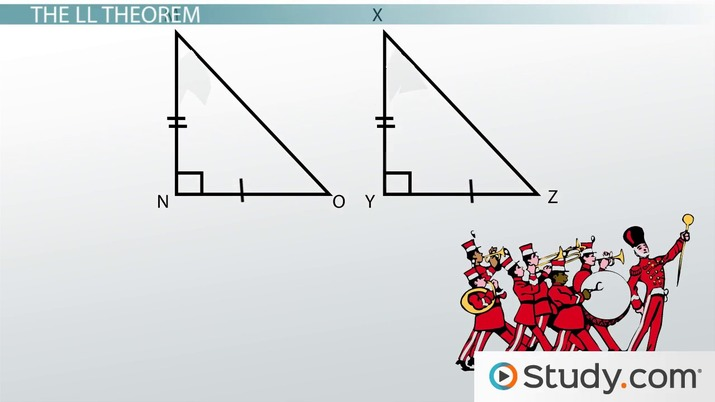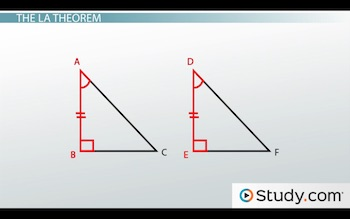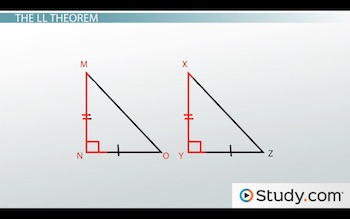Math Courses / Course / Chapter

# Congruency of Right Triangles: Definition of LA and LL Theorems

Lesson Transcript
Instructor: Jeff Calareso

Jeff teaches high school English, math and other subjects. He has a master's degree in writing and literature.

Two theorems useful to proving whether right triangles are congruent are the leg-acute (LA), and leg-leg (LL) theorems. Learn about the features of right triangles and how to use the LA and LL theorems to establish congruence between two right triangles. Updated: 09/29/2021

## Right Triangles

Right triangles aren't like other, ordinary triangles. Ordinary triangles just have three sides and three angles. They're like the random people you might see on a street. They can be tall and skinny or short and wide. There's no order or consistency.

Right triangles are consistent. They always have that clean and neat right angle. They're like a marching band. Sure, there are drummers, trumpet players and tuba players. But they all have those same uniforms, and they march in organized patterns.

And think about the uniform of a right triangle. There's the hypotenuse. It's always the longest side, and it's always in the same place, opposite the 90 degree angle. Then there are the legs. These are the other two sides. They meet to form the 90-degree angle.

When we're trying to prove congruency with triangles, right triangles like to save us a step, so to speak. Other triangles require three matches. There's the side-angle-side postulate, the angle-side-angle postulate and others. Right triangles say, 'Hey, we're already in uniform and in a line. We'll help you out.'An error occurred trying to load this video.

Try refreshing the page, or contact customer support.

Coming up next: Congruency of Isosceles Triangles: Proving the Theorem

### You're on a roll. Keep up the good work!

Replay
Your next lesson will play in 10 seconds
• 0:01 Right Triangles
• 1:10 The LA Theorem
• 2:59 The LL Theorem
• 4:07 Using the Theorems
• 6:11 Lesson Summary
Save Save

Want to watch this again later?

Timeline
Autoplay
Autoplay
Speed Speed

## The LA Theorem

Let's start with the LA theorem. The LA Theorem is my theory about how Los Angeles traffic is, wait, hang on...this LA has nothing to do with Los Angeles. The LA in LA theorem refers to leg-acute. It states that if the leg and an acute angle of one right triangle are congruent to the corresponding leg and acute angle of another right triangle, then the triangles are congruent.

Whew. That theorem takes about as long to explain as it takes to get anywhere in LA traffic. I'm pretty sure marching bands would move more quickly on the 405. As for the LA theorem, when you think about it, it's very logical.

Let's look at two triangles, ABC and DEF.They definitely look like they belong in a marching band together, don't they? We know that they're both right triangles. Angles B and E are each 90 degrees. And we know AB is congruent to DE and angle A is congruent to angle D.

Using the LA theorem, we could say, 'Ok, two right triangles. And we've got a leg and an acute angle that match, so they're congruent.' But why is this true?

Notice that since B and E are congruent, too, that this is really just the angle-side-angle postulate. The fact that they're right triangles just gives us a shortcut. It's like being born with a trumpet already in your hands. Hmm, maybe that's not a good idea.

But even if we didn't have included sides, like AB and DE here, it would still be just like ASA. What if we know A and D are congruent, but then BC and EF? Well, since the sum of the interior angles of a triangle is 180, we know that C and F must also be congruent to each other. So we still get our angle-side-angle.

## The LL Theorem

Next, let's talk about the LL theorem. The LL Theorem is about a Maine-based sporting goods retailer...wait, no. That's LL Bean. Different thing. The LL theorem is the leg-leg theorem. LA theorem is leg-acute, so it makes sense that LL is leg-leg. It states that if the legs of one right triangle are congruent to the legs of another right triangle, then the triangles are congruent.

This is like marching bands with their matching pants. Their legs look alike, right?

Below are two triangles: MNO and XYZ.Maybe MNO plays the trombone and XYZ plays the xylophone. We know they're right triangles. Angles N and Y are each 90 degrees. If we know that MN is congruent to XY and NO is congruent to YZ, then we have two legs. This enables us to say they're congruent based on the LL theorem.

The LL theorem is really just the SAS postulate, or side-angle-side. Remember that the legs of a right triangle always meet at a right angle, so we always know the included angle between them.

To unlock this lesson you must be a Study.com Member.

### Register to view this lesson

Are you a student or a teacher?

Back

### Resources created by teachers for teachers

Over 30,000 video lessons & teaching resources‐all in one place.Video lessonsQuizzes & WorksheetsClassroom IntegrationLesson Plans

I would definitely recommend Study.com to my colleagues. It’s like a teacher waved a magic wand and did the work for me. I feel like it’s a lifeline.

Jennifer B.
TeacherCreate an account to start this course today
Used by over 30 million students worldwide